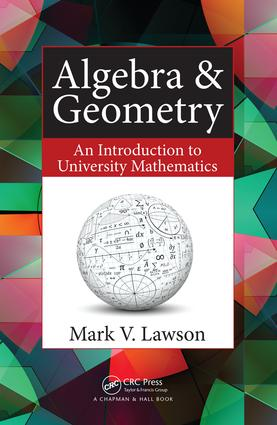# Algebra & Geometry

## An Introduction to University Mathematics, 1st Edition

Chapman and Hall/CRC

384 pages | 89 B/W Illus.

Paperback: 9781482246476
pub: 2016-05-12
SAVE ~\$13.99
\$69.95
\$55.96
x
Hardback: 9781138401693
pub: 2017-07-27
SAVE ~\$41.00
\$205.00
\$164.00
x
eBook (VitalSource) : 9781315381978
pub: 2016-11-25
from \$33.98

FREE Standard Shipping!

### Description

Algebra & Geometry: An Introduction to University Mathematics provides a bridge between high school and undergraduate mathematics courses on algebra and geometry. The author shows students how mathematics is more than a collection of methods by presenting important ideas and their historical origins throughout the text. He incorporates a hands-on approach to proofs and connects algebra and geometry to various applications.

The text focuses on linear equations, polynomial equations, and quadratic forms. The first several chapters cover foundational topics, including the importance of proofs and properties commonly encountered when studying algebra. The remaining chapters form the mathematical core of the book. These chapters explain the solution of different kinds of algebraic equations, the nature of the solutions, and the interplay between geometry and algebra

### Reviews

"This book is for anyone who, having had a taste of higher school mathematics, wants to see what serious math at a university level is truly like. It introduces all the important topics in algebra and algebra as it affects geometry and does so in a totally uncompromised fashion. Professor Lawson shows respect for the subject through the patience exercised in developing the material thoroughly while keeping the reader engaged with a conversational style that never loses clarity. Along the way, there are some real novelties that will surprise even seasoned readers of mathematics."

—Professor Peter M. Higgins, F.I.M.A., University of Essex

IDEAS

The Nature of Mathematics

MATHEMATICS IN HISTORY

MATHEMATICS TODAY

THE SCOPE OF MATHEMATICS

WHAT THEY (PROBABLY) DIDN’T TELL YOU IN SCHOOL

Proofs

MATHEMATICAL TRUTH

FUNDAMENTAL ASSUMPTIONS OF LOGIC

FIVE EASY PROOFS

AXIOMS

UN PETIT PEU DE PHILOSOPHIE

MATHEMATICAL CREATIVITY

PROVING SOMETHING FALSE

TERMINOLOGY

Foundations

SETS

BOOLEAN OPERATIONS

RELATIONS

FUNCTIONS

EQUIVALENCE RELATIONS

ORDER RELATIONS

QUANTIFIERS

PROOF BY INDUCTION

COUNTING

INFINITE NUMBERS

Algebra Redux

THE RULES OF THE GAME

ALGEBRAIC AXIOMS FOR REAL NUMBERS

THE BINOMIAL THEOREM

BOOLEAN ALGEBRAS

CHARACTERIZING REAL NUMBERS

THEORIES

Number Theory

THE REMAINDER THEOREM

GREATEST COMMON DIVISORS

THE FUNDAMENTAL THEOREM OF ARITHMETIC

MODULAR ARITHMETIC

CONTINUED FRACTIONS

Complex Numbers

COMPLEX NUMBER ARITHMETIC

COMPLEX NUMBER GEOMETRY

EULER’S FORMULA

MAKING SENSE OF COMPLEX NUMBERS

Polynomials

TERMINOLOGY

THE REMAINDER THEOREM

ROOTS OF POLYNOMIALS

THE FUNDAMENTAL THEOREM OF ALGEBRA

ARBITRARY ROOTS OF COMPLEX NUMBERS

GREATEST COMMON DIVISORS OF POLYNOMIALS

IRREDUCIBLE POLYNOMIALS

PARTIAL FRACTIONS

ALGEBRAIC AND TRANSCENDENTAL NUMBERS

MODULAR ARITHMETIC WITH POLYNOMIALS

Matrices

MATRIX ARITHMETIC

MATRIX ALGEBRA

SOLVING SYSTEMS OF LINEAR EQUATIONS

DETERMINANTS

INVERTIBLE MATRICES

DIAGONALIZATION

BLANKINSHIP’S ALGORITHM

Vectors

VECTORS GEOMETRICALLY

VECTORS ALGEBRAICALLY

THE GEOMETRIC MEANING OF DETERMINANTS

GEOMETRY WITH VECTORS

LINEAR FUNCTIONS

THE ALGEBRAIC MEANING OF DETERMINANTS

QUATERNIONS

The Principal Axes Theorem

ORTHOGONAL MATRICES

ORTHOGONAL DIAGONALIZATION